﻿ 基于动态曲线的车道检测算法

# 基于动态曲线的车道检测算法Lane Detection Algorithm Based on Dynamic Curve

Abstract: Aiming at the detection of complex lanes in unmanned driving technology, this paper proposes a complex lane detection algorithm based on the color gradient change of lanes and the correlation of each video frame. The algorithm extracts the edge pixels of the original image by gradient detection method, extracts the feature points of the edge pixels through the restriction of dynamic curve, filters out the target edge pixels and removes the non-target edge pixels. Finally, the lane location is detected by curve fitting. Field measurements show that the algorithm can finally obtain more accurate location information of complex lane lines through curve fitting. At the same time, the measured results also show that this algorithm has better real-time performance and robustness than other algorithms.

1. 引言

2. 本文提出的车道检测算法

2.1. 车道检测基本原理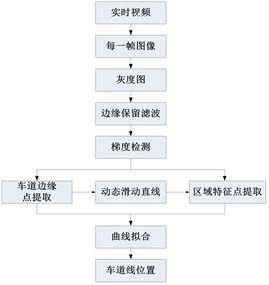Figure 1. Basic principle of lane detection

2.2. 相机标定

2.3. 边缘保留滤波

${W}_{{\sigma }_{s}}={\text{e}}^{-\frac{{\left({x}_{i}-{x}_{c}\right)}^{2}+{\left({y}_{i}-{y}_{c}\right)}^{2}}{2{\sigma }^{2}}}$ (1)

${W}_{{\sigma }_{r}}={\text{e}}^{-\frac{{\left(gray\left({x}_{i,}{y}_{i}\right)-gray\left({x}_{c}-{y}_{c}\right)\right)}^{2}}{2{\sigma }^{2}}}$ (2)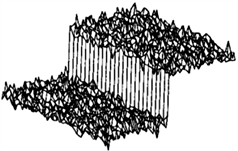(a)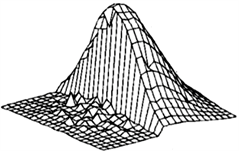(b)(c)

Figure 2. Edge preserving filtering principle diagram

2.4. 梯度检测

${G}_{x}=\left[\begin{array}{ccc}-1& 0& +1\\ -2& 0& +2\\ -1& 0& +1\end{array}\right]$ (3)(a)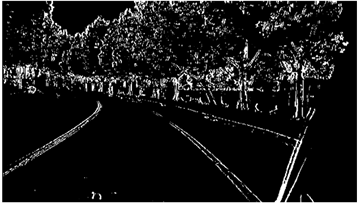(b)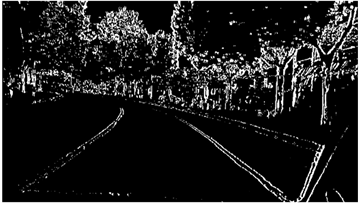(c)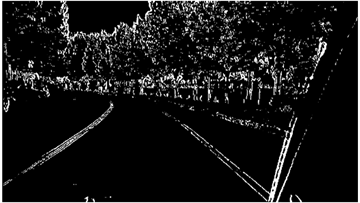(d)

Figure 3. Two valued image after mathematical morphology processing

2.5. 特征点及曲线拟合

$x=A{y}^{2}+By+C$ (4)

$x=A{y}^{2}+By+C±D$ (5)

3. 实验结果分析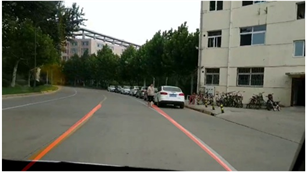(a)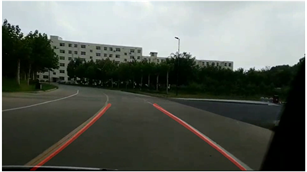(b)(c)(d)

Figure 4. Lane detection result diagram

4. 结论

 Wang, Y., Teoh, E.K. and Shen, D. (2004) Lane Detection and Tracking Using B-Snake. Image & Vision Computing, 22, 269-280.
https://doi.org/10.1016/j.imavis.2003.10.003

 Borkar, A., Hayes, M. and Smith, M.T. (2010) Robust Lane Detection and Tracking with RANSAC and Kalman Filter. IEEE International Conference on Image Processing, Cairo, 7-10 November 2009, 3225-3228.

 朱彤, 阎莹, 袁志业. 一种基于形态学变换的车道检测方法[J]. 山东交通学院学报, 2006, 14(1): 16-19.

 陈勇, 黄席樾, 唐高友, 等. 基于机器视觉的车道检测与二维重建方法[J]. 仪器仪表学报, 2007, 28(7): 1205-1210.

 林国余, 陈旭, 张为公. 基于多信息融合优化的鲁棒性车道检测算法[J]. 东南大学学报(自然科学版), 2010, 40(4): 771-777.

 李明, 黄华, 夏建刚. 基于Hough变换的车道检测改进算法研究[J]. 计算机工程与设计, 2012, 33(4): 1638-1642.

 Cheng, H.-Y., Jeng, B.-S., Tseng, P.-T., et al. (2006) Lane Detection with Moving Vehicles in the Traffic Scenes. IEEE Transactions on Intelligent Transportation Systems, 7, 571-582.
https://doi.org/10.1109/TITS.2006.883940

 Zhou, Y., Xu, R., Hu, X., et al. (2006) A Robust Lane Detection and Tracking Method Based on Computer Vision. Measurement Science & Technology, 17, 736.
https://doi.org/10.1088/0957-0233/17/4/020

 Liu, W., Wen, X.Z., Duan, B., et al. (2007) Rear Vehicle Detection and Track-ing for Lane Change Assist. Intelligent Vehicles Symposium, Istanbul, 13-15 June 2007, 252-257.
https://doi.org/10.1109/IVS.2007.4290123

 Borkar, A., Hayes, M. and Smith, M. (2009) Lane Detection and Tracking Using a Layered Approach. Lecture Notes in Computer Science, 5807, 474-484.
https://doi.org/10.1007/978-3-642-04697-1_44

 Zhang, Z. (2000) A Flexible New Technique for Camera Calibration. IEEE Transactions on Pattern Analysis and Machine Intelligence, 22, 1330-1334.
https://doi.org/10.1109/34.888718

 刘权, 吴陈, 潘舒, 等. 边缘保留的图像滤波方法[J]. 微计算机信息, 2007, 23(9): 309-310.

 唐继勇. 基于边缘保持滤波的Canny彩色图像边缘检测方法[J]. 现代电子技术, 2012, 35(11): 81-83.

 徐勇. 边缘结构保持型的图像滤波算法研究[D]: [硕士学位论文]. 合肥: 合肥工业大学, 2011.

Top